Next: Finite slit: Diffraction Up: N narrow slits Previous: Analysis

### Interpretation

Again, several features of our result, Eq. 17, are noteworthy. Figure 7 shows this result for a case with N=6 slits.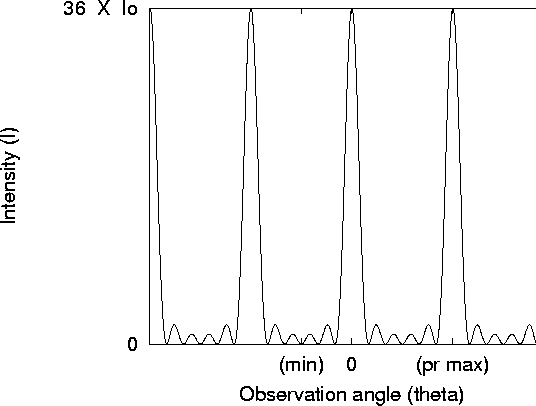Figure 7: Intensity as a function of observation angle for an N-slit experiment for the case N=6. In this case, principle maxima appear in the location of every sixth minima, so that there are five minima between principle maxima, and thus four lesser maxima between principle maxima.

• Case of two slits: If we substitute N=2 into Eq. 17 and use the double-angle sine formula, we have for the numerator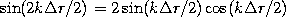, so that the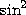term in the denominator cancels, leaving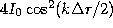, precisely the two-slit result, Eq. 13.
• Principle maxima: we find extremely large maxima whenever the denominator in Eq. 13 goes to zero. This occurs whenever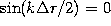, equivalently,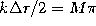, where M is some integer. This means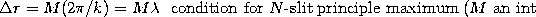so that the path difference between all of the slits is always an exact number of wavelengths, leading to complete constructive interference. To see how large the maxima can be, we take the limit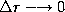. From the small-angle formula, we then have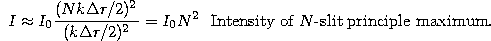The physical reason for the factor of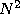is just that the amplitudes of all N waves add, so the amplitude is N times as large, but the intensity, of course, goes like the amplitude squared.

• Minima: Minima occur in the pattern whenever the numerator is zero (and the denominator is not). Zeros in the numerator occur whenever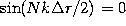, equivalently,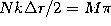, where M is any integer, so that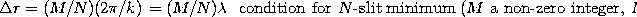Note that the spacing between minima is thus exactly 1/N times the spacing between principle maxima. Often, you can use this to determine the number of slits from the appearance of the diffraction pattern.

• Lesser maxima: Of course, there are other maxima which must appear approximately halfway between neighboring minima, the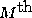of these happens where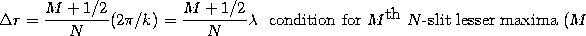Next: Finite slit: Diffraction Up: N narrow slits Previous: Analysis

Tomas Arias
Thu Sep 13 15:26:14 EDT 2001